Getting to E5 With Ease: An AltBOC Double-Sideband Receiver Based on Single-Sideband Correlation - Inside GNSS - Global Navigation Satellite Systems Engineering, Policy, and Design

# Getting to E5 With Ease: An AltBOC Double-Sideband Receiver Based on Single-Sideband Correlation

A novel method to track Galileo E5 AltBOC signal phase adjusts and then combines the correlation results of the upper sideband and lower sideband signal to form a double-sideband correlation result, which is equivalent to AltBOC wideband receiving to some extent. Thus, a Galileo E5 wideband receiver can be achieved with only minor modification to a traditional GNSS single-sideband receiver. The method can be further applied to other binary offset carrier (BOC) signals.

JUN MO, TISHENG ZHANG, AND XIAOJI NIU

AUTHORS FROM WUHAN UNIVERSITY AND GLOBSKY TECHNOLOGY INC.

The E5 signal is currently the most complex one of all Galileo signals; and it is also the GNSS signal with the largest spectrum bandwidth. Its total bandwidth is 51.15MHz, with two single-sided main lobe bandwidths at 20.46MHz and 30.69MHz apart. The wideband signal has the advantages of strong anti-multipath capability and high observation accuracy.

A modified version of a binary offset carrier (BOC), Galileo E5 has code rate of 10.23 MHz and a sub-carrier frequency of 15.345 MHz. AltBOC(15,10) is a wideband signal transmitted at 1191.795 MHz. The four signal components that it carries (E5a-I, E5a-Q, E5b-I and E5b-Q) are combined into a signal with constant envelope characteristics. The frequency spectrum is dispersed on the two closely located frequency bands E5a and E5b.

For a receiver, the E5 signal modulated by AltBOC can be treated as two independent single sideband signals, E5a and E5b, and each sideband signal is modulated by data and pilot channel through QPSK modulation. To avoid using a wideband RF front end, the receiver can independently process the upper and lower sideband signals with a BPSK-like method. The single-side narrowband processing method not only maintains compatibility with the processing architecture of other GNSS signals, but also reduces the signal processing complexity. However, at the same time, it loses its advantages of anti-multipath and observation accuracy.

To fully utilize the advantages of the AltBOC wideband signal, the receiver needs to process the entire signal as a whole. In implementation, whether the sub-carrier and pseudo-code are processed separately or together, the bandwidth of the signal to be processed must cover the entire E5 signal [1-3]. Both the wide bandwidth and the unique structure of the AltBOC signal increase the complexity of baseband signal processing and reduce the design compatibility with other GNSS signals .

To solve the complexity and compatibility issues of signal processing while maintaining the performance advantages of AltBOC broadband signals, a double sideband combined demodulation method is proposed, based on the analysis of the characteristics of AltBOC signal. This method can simultaneously have the advantages of simple processing in single-sideband signal reception and the advantages of strong anti-multipath capability and high observation accuracy in double-sideband signal reception.

### Correlation Characteristics of Galileo E5 signal

The Galileo E5 signal consists of two intrinsic signal components, E5a and E5b. The E5a signal consists of the data signal component eE5a-I(t) modulated on the I channel and the pilot signal component eE5a-Q(t) modulated on the Q channel. Similarly, E5b has the data signal component eE5b-I(t) modulated on the I channel and the pilot signal component eE5b-Q(t) modulated on the Q channel. Each signal component can be regarded as a binary phase shift keying (BPSK) modulated signal. Therefore, the Galileo E5 signal sE5(t) is obtained by AltBOC modulation of four binary signal components, and its expression is :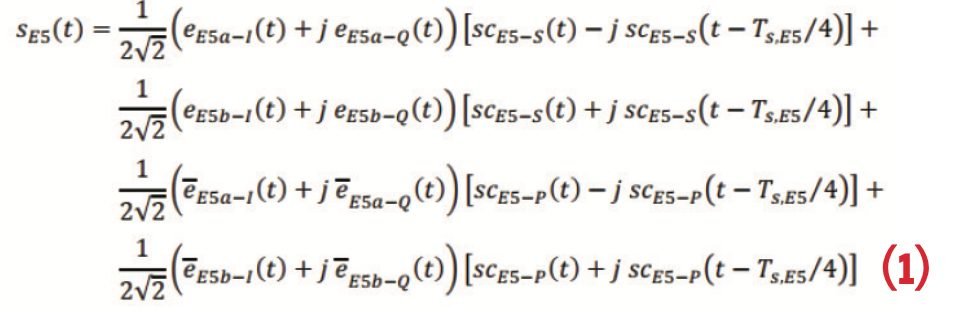scE5-S(t) and scE5-P(t) are two subcarriers with four levels, the frequency of the subcarrier is RS,E5 = 1/TS,E5 = 15.345MHz (15 x 1.023MHz).

The sE5(t) calculated by this mathematical expression can also be equivalently expressed as an 8PSK signal with different phase modulation. The upper part of Table 1 completely lists 16 combinations of four binary signal component values, and the lower part lists the AltBOC modulation value of each input value combination in 8 time slots of a subcarrier period. The formula and the look-up table are equivalent. Both can be used to generate Galileo E5 signal while the look-up table method is simpler.

There are two methods to do correlation on Galileo E5 signals. The first one is double sideband signal correlation, which is to process E5 signal as a whole complex signal. The second one is single sideband signal correlation. In this method, the E5 signal is divided into two sidebands, E5a and E5b, and each sideband is treated as an ordinary QPSK (10) signal and processed separately.

When doing the double sideband signal correlation of the Galileo E5 signal, the input signal follows Equation (1) after removing the carrier and Doppler. The local signal correlated with the input signal is the conjugate of the aforementioned baseband signal. With this method, the shape of the correlation peak is shown in Figure 1. It has one main peak and multiple sub peaks. Since the main peak with the highest energy is very narrow, it has much better pseudo code tracking accuracy and better multipath mitigation effect.

Using QPSK demodulation method to do correlation on AltBOC signal with four PRN code respectively, four triangular shaped correlation peaks can be obtained as shown in Figure 2. It is consistent with the traditional QPSK correlation peak. Its tracking accuracy and multipath suppression ability are also similar to traditional QPSK signals. So the potential advantages of the AltBOC signal in terms of accuracy and multipath suppression cannot be fully utilized.

The AltBOC signal modulates the four signal components together through the carrier and subcarriers, and the four signal components have certain phase relationship. When the subcarriers are stripped and the PRN codes of the four signal components are used to correlate with AltBOC, the respective phase information of the correlation results is shown in Figure 3. The phase of the correlation value obtained by the correlation of the E5aI signal is -π/8, and the phase of the correlation value obtained by the correlation of the E5bI signal is π/8. The phase of the correlation value of the corresponding Q branch signal is ahead of the phase of the correlation value of the I branch signal by π/2. The double sideband correlation implicitly utilizes this internal relationship of the four signal components, while the information is lost in the single sideband correlation process.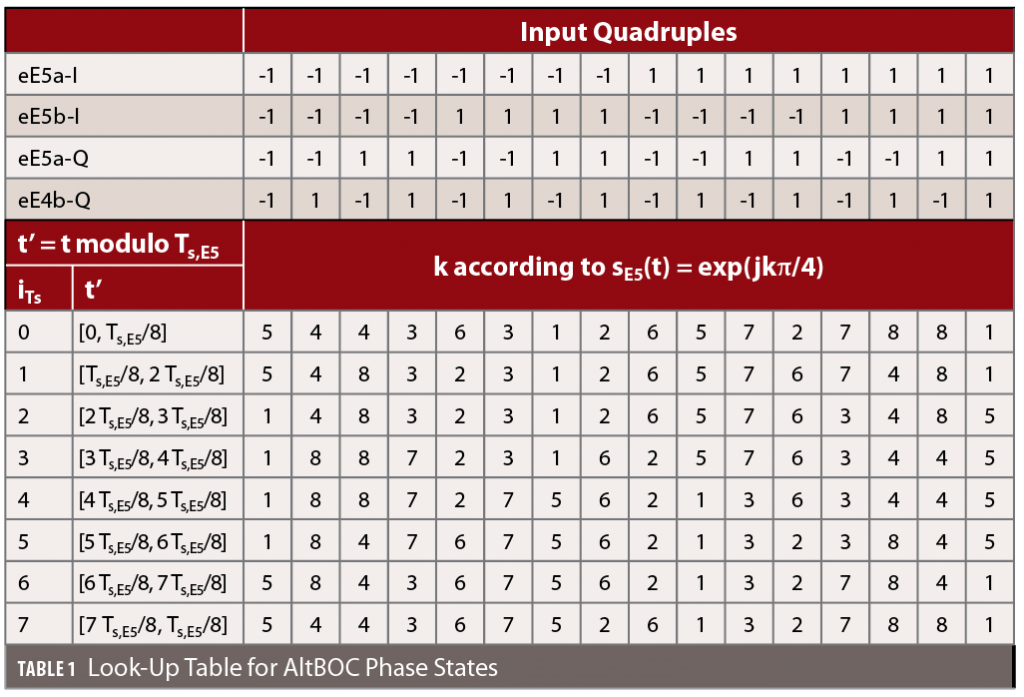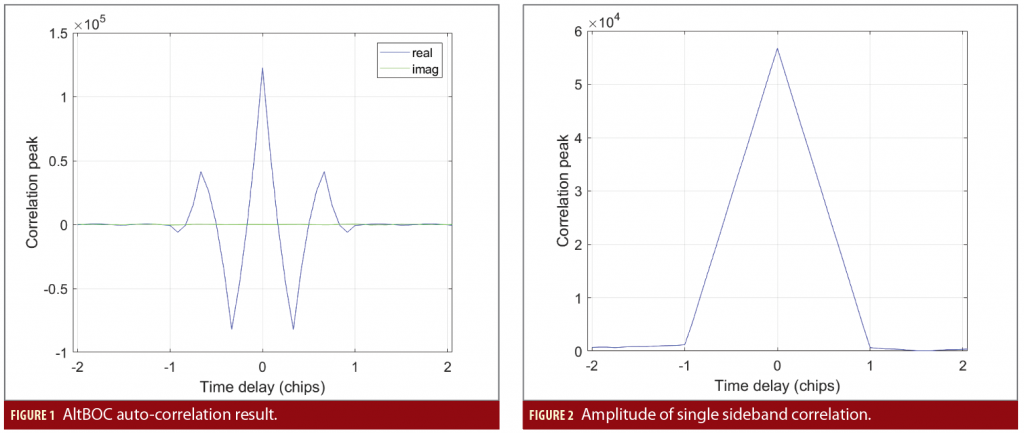### Double sideband combined demodulation

According to the two correlation methods of the AltBOC signal in the previous section, the double sideband correlation processing makes full use of the advantages of the AltBOC signal, but requires a wider bandwidth, a higher sampling rate, complex signal processing strategy and a poor compatibility with traditional signal processing architecture. While the single sideband correlation processing is similar to the traditional QPSK signal strategy, but the correlation result loses the phase information and cannot use the advantages of the AltBOC signal. Considering that the energy of the AltBOC signal is mainly concentrated on the two side lobes, the idea of multi-carrier modulation (MCM) in communication can be adopted to process the two sidebands separately and then combine them together. The specific implementation procedure is to treat the two side lobes as sub-bands when doing correlation first, and then taking into account the phase relationship between the sub-band correlation results, restore the double sideband correlation results by combining the correlation results of different code delays in the sub-bands, namely double sideband combined demodulation method. The following describes the double sideband combined demodulation method in detail. The AltBOC signal can be treated as two QPSK signals distributed on different frequencies when being tracked, and these two QPSK signals have certain phase relationship. Therefore, we can construct a superimposed signal with two QPSK signals with different subcarriers. This signal modulates the two PRN codes of E5a and the two PRN codes of E5b with the frequencies of two subcarriers, and the two modulation carriers also has certain phase relationship similar to AltBOC signal. Simplified, we name this signal QBOC signal. The mathematical expression of QBOC baseband complex signal is as follows: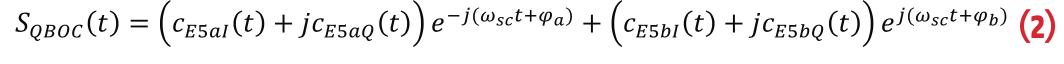In which cE5aI, cE5aQ, cE5bI, and cE5bQ represent the modulated PRN codes modulated on the four frequency components (data modulation is ignored). φa and φb are the initial phases of the two sub-carriers. In the AltBOC signal, these two phases are behind and ahead by π/8 respectively, so π/8 is adopted in QBOC accordingly.

The QBOC signal and the AltBOC signal have very similar power spectrum, the same average energy, and the four signal components have the same phase relationship. The difference between them is that the AltBOC signal has a constant envelope while the QBOC signal is not. This difference does not affect the correlation characteristics of the signal. We can use the QBOC signal instead as the local signal to correlate with the input AltBOC signal. The correlation result between the input signal (which is AltBOC signal) and the local QBOC signal can be expressed as:

Within which s*QBOC(t) is the conjugate of QBOC signal. Figure 4 is the cross correlation result between QBOC and AltBOC. Comparing Figure 4 and Figure 1, it can be found that the cross correlation result is very similar to the auto-correlation result of AltBOC signal.Putting equation (2) into equation (3), we can get: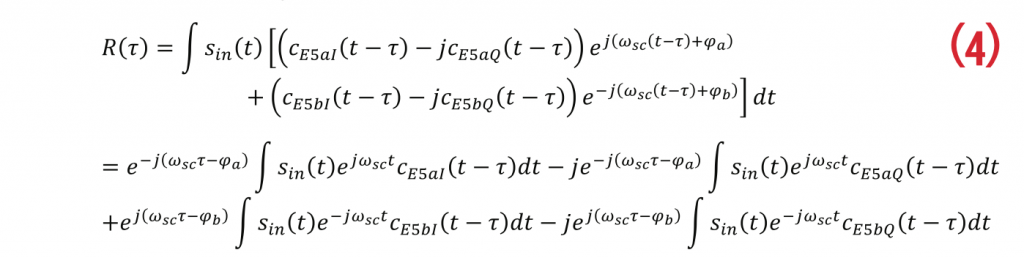Analyzing the above equation, it can be found that after being divided into four integral terms, the content of the integral part is equivalent to the correlation result of correlation of the PRN code with the input signal with sinusoidal form of subcarrier removed. That is to say, the correlation result can be considered as the result of following procedures: demodulate the input AltBOC signal with sub-carrier first, then do correlation as a single sideband signal respectively, and add the four single sideband correlation results together with phase adjusted finally. Therefore, the equivalent correlation result of the complete AltBOC signal can be expressed as a combination of the correlation results of the single sideband QPSK signal, or be expressed in the following form: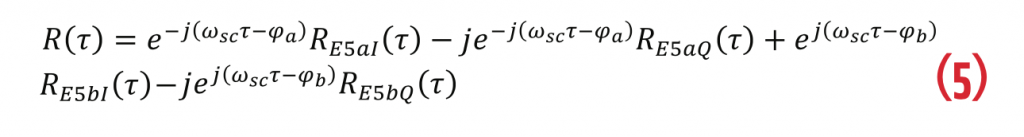While tracking the signal, the receiver can only track the pilot channel. That is to say, only Q branch relation result is preserved. Then the double sideband combined result can be expressed as:The above analysis shows that when the receiver performs single sideband correlation processing separately according to the traditional BPSK/QPSK signal processing method, and then phase-adjusts the correlation results and adds them together, the complete double sideband correlation result can be restored. The method will be verified by in the following section.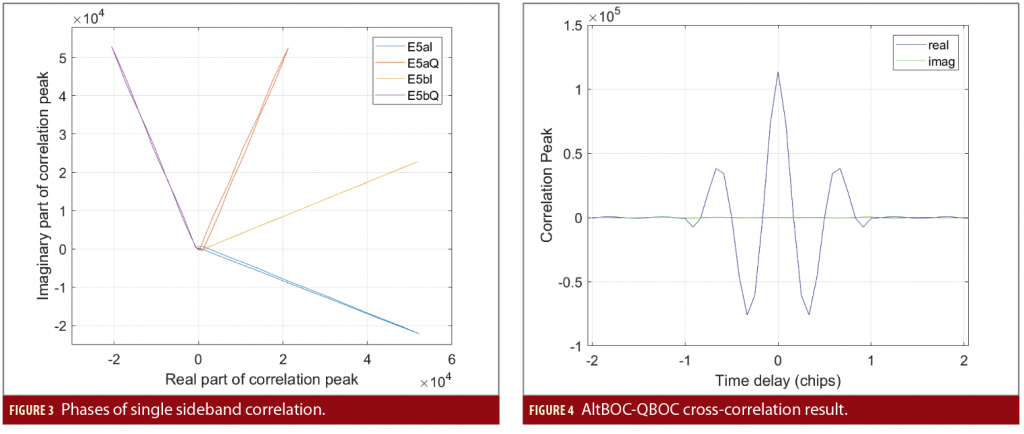### Verification of Double-Sideband Demodulation

In the actual narrow band Galileo receiver with wideband radio-frequency (RF) front-end, the RF front-end output wideband intermediate frequency (IF) sampling data containing the complete AltBOC signal, and then the signal is up/down converted, filtered, and down-sampled to separate narrow-band signals containing upper and lower sidebands signal respectively. Without loss of generality, we use the sampling rate of 88MHz and the local oscillator (LO) frequency in RF is 1192MHz (the corresponding nominal intermediate frequency is -0.205MHz) as an example to analyze the baseband signal processing.

Figure 5 shows the implementation of double-sideband combined demodulation. First, a signal with a sampling rate of 8 times the subcarrier frequency (122.76MHz) is generated using the look-up table method, and then it is resampled to 88MHz to simulate the digital IF output of the RF front-end. The subsequent processing procedure is consistent with the traditional QPSK signal baseband processing procedure in the narrow band Galileo receiver. After two independent down-conversions with known initial phases, the upper and lower sidebands are converted to zero IF respectively, and then filtered and down-sampled to 22MHz. These narrow band upper and lower sideband signals are processed by correlation operations.

At this time, the correlation result of the triangular peak shape can be obtained. To restore the complete double-sideband correlation results of AltBOC, on the basis of the above-mentioned traditional baseband signal processing, the corresponding phase adjustments are performed on the upper and lower sideband correlation results, and the coherent accumulation is performed. Because additive thermal noise is considered to be random and has average value of zero, the thermal noise is not added to the signal to make the results clearer.

According to the simulation results of AltBOC signal generation and baseband processing with the procedure given in Figure 5, the analysis of the result is shown as below. The shape of the amplitude of the single sideband correlation is shown in Figure 6. It is a triangle with a flattened top, which is consistent with the correlation result of the QPSK signal. And the phases of the correlation results are shown in Figure 7. The interval of the correlator is 1/8 chip.

Set τ=0 for the peak correlator and appreciate value of τ on other correlators with their delay, then put the result into the equation (6) to obtain the real and imaginary parts of the correlation value shown in Figure 8. The shape of the correlation peak of the correlation result is basically the same as the double-sideband correlation result of the AltBOC signal in Figure 4, only the top of the correlation peak becomes flatter due to the influence of the band-limit filter.

In a real receiver, when the signal is up-converted, the initial phases of the up-conversion signal for E5a and the down-conversion signal of E5b cannot be guaranteed to be zero at the beginning of every code period. Therefore, there must be additional circuit to help us get the initial phases. Once the initial phases are obtained, for example 120 degrees and -75 degrees in our simulation respectively, the phase relationship of the correlation results of the 4 component signals is shown in Figure 9. The I/Q branches of E5a and E5b are still orthogonal, but their relative values are different from those in Figure 7. This is because the local signal used for up-
down conversion has an initial phase superimposed on the correlation result. After compensating the correlation result by removing the initial phases respectively, the results are consistent with
Figure 7.

Simulation results show that adopting traditional single sideband correlation processing, and then performing phase adjustment and accumulation, a complete double sideband correlation result can be obtained.

### Verification with Ionospheric Delay

Since Galileo’s E5 signal is a wideband signal with the distance between the two main lobes over 30 MHz and the bandwidth of each side lobe over 20MHz, the total bandwidth of E5 signal exceeds 50MHz. The in-band dispersion cannot be ignored when the signal passes through the ionosphere. The magnitude of the ionospheric delay is related to the number of free electrons, and the index used to measure it is Total Electron Content (TEC). When signals of different frequencies pass through the ionosphere, their phase delay and group delay can be approximated by the following equations: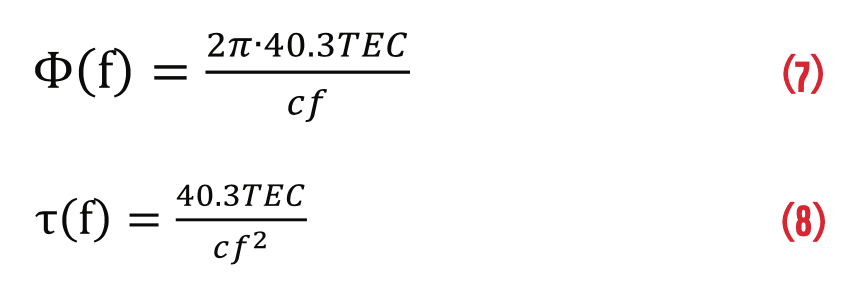The above analysis shows that when the receiver performs single sideband correlation processing separately according to the traditional BPSK/QPSK signal processing method, and then phase-adjusts the correlation results and adds them together, the complete double sideband correlation result can be restored. The method will be verified by in the following section.

Figure 10 and Figure 11 show the correlation results of the upper and lower sidebands when TEC=0 and TEC=1. At TEC=1, due to the dispersion caused by the ionosphere, the phase of the correlation result has changed significantly, and the phase difference between the upper and lower sidebands also changed. Figure 12 shows the phase of the correlation result of TEC=10. At this time, the phase of the correlation result of E5aI has changed from lagging behind E5bI to ahead of E5bI.

Figure 13 shows the AltBOC wideband correlation results and Figure 14 shows the correlation results using the double sideband combined method. For different ionospheric delays, the combined correlation results still conform to the characteristics of the correlation peak of the AltBOC wideband correlation results.

With the increase of TEC, the delay of the correlation peak increases gradually, reflecting the group delay caused by the ionosphere. The correlator interval is 1/12 chip. It can be seen from the figure that when TEC=8, the group delay is about 1/12 chip behind, which is consistent with the ionospheric group delay at E5 center frequency (2.27m) based on theoretical calculation. The above analysis results show that, in the presence of ionospheric dispersion, the double-sideband combined demodulation method can also be applied.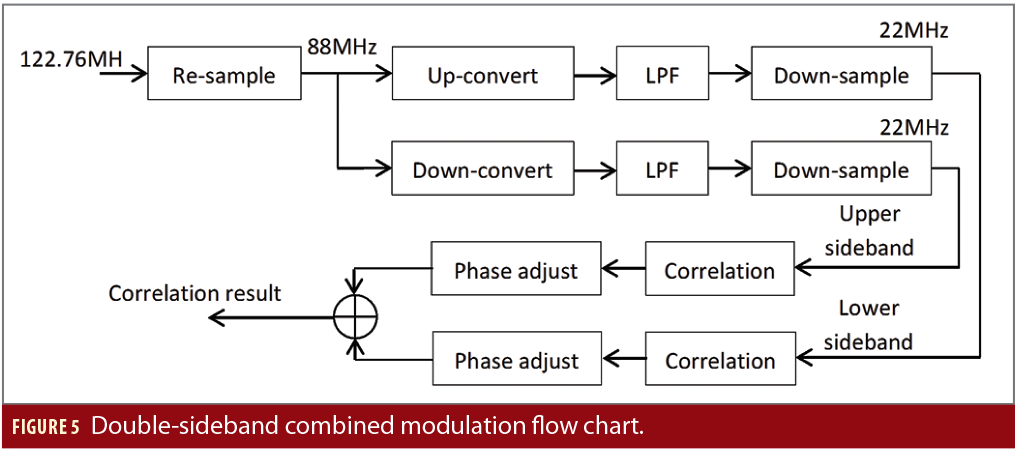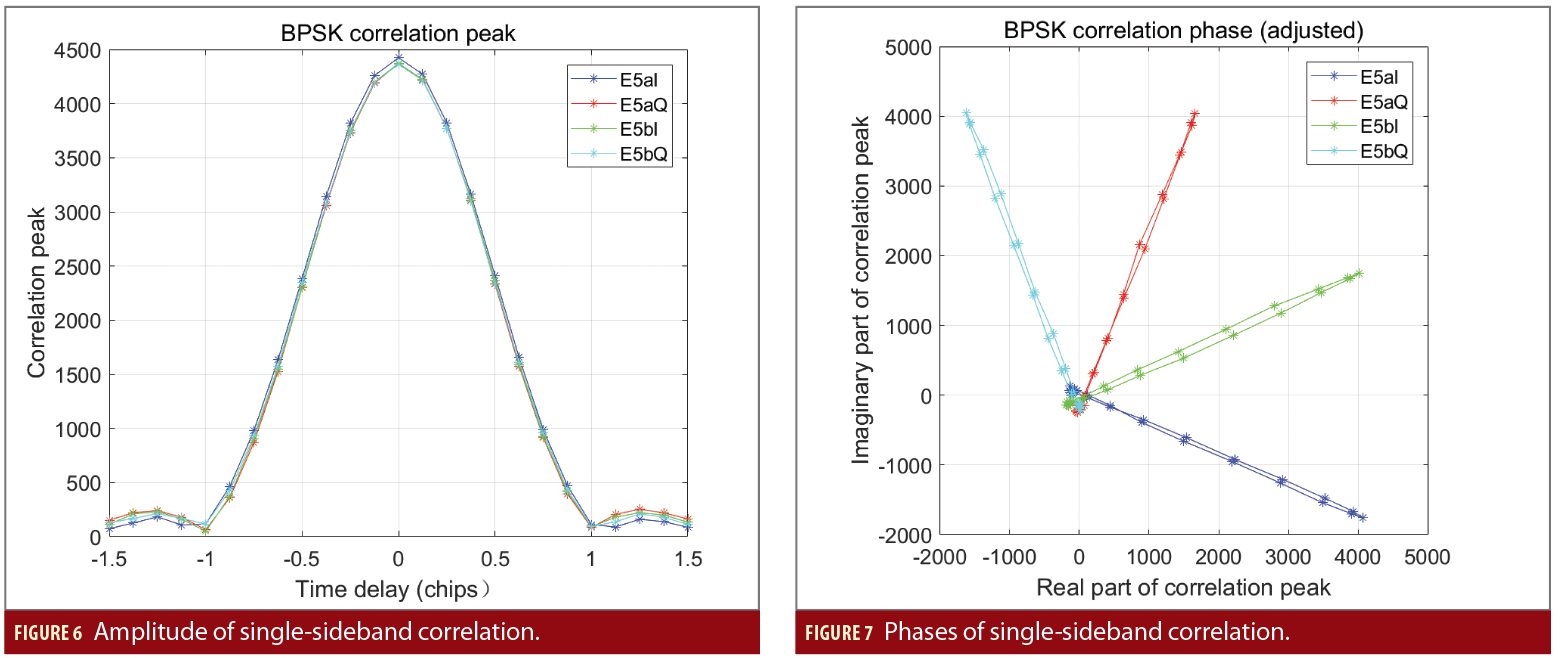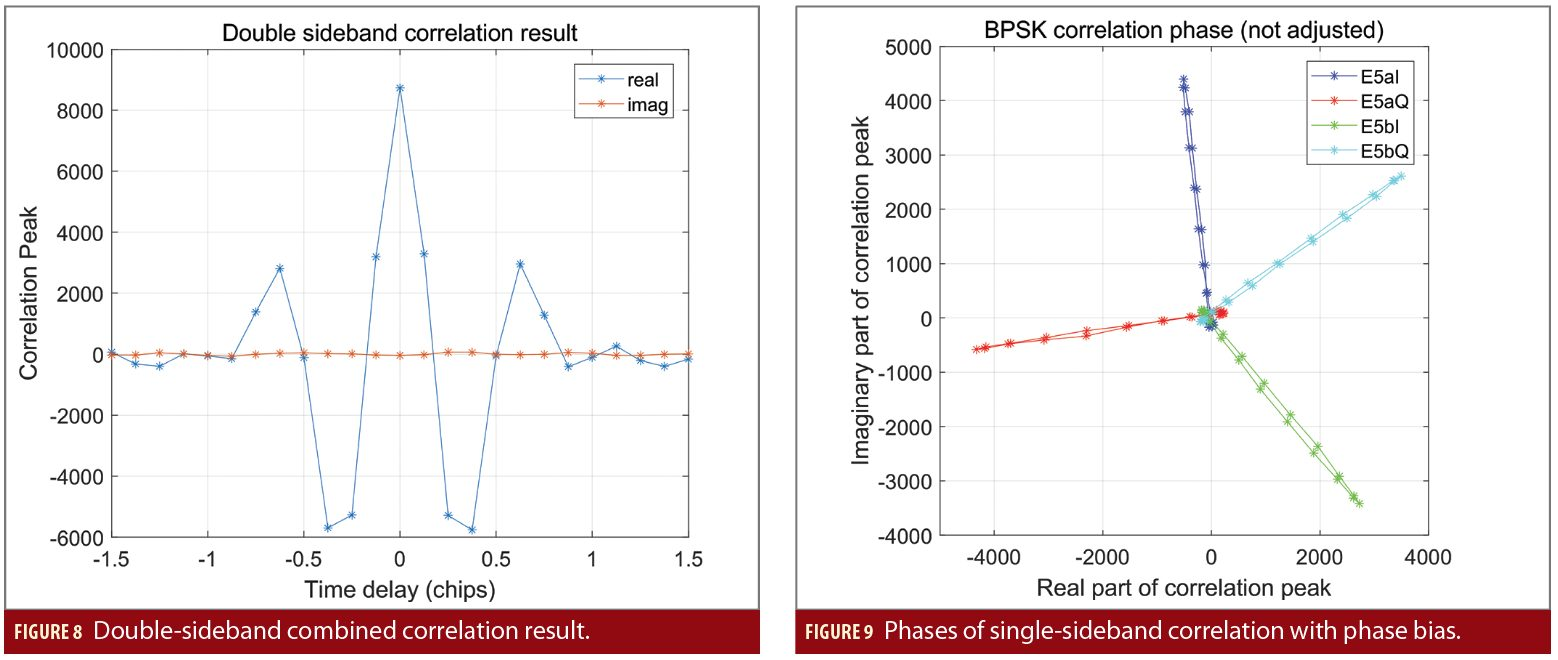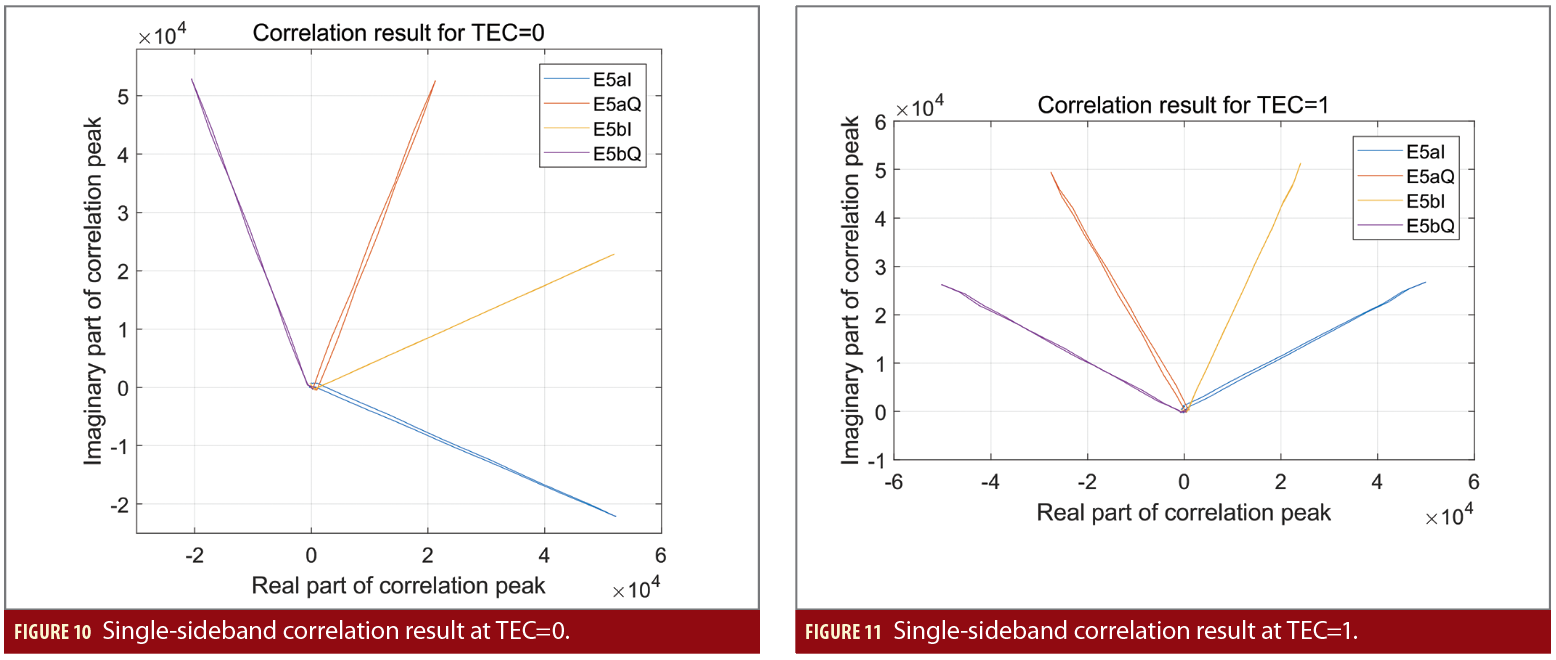### Conclusion

Future work will focus on the real AltBOC signal processing for experimental test verification of the proposed method, and further extend it to other BOC signals.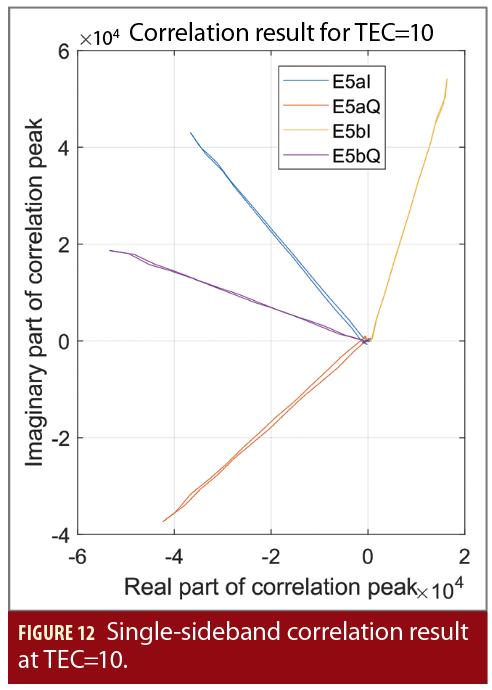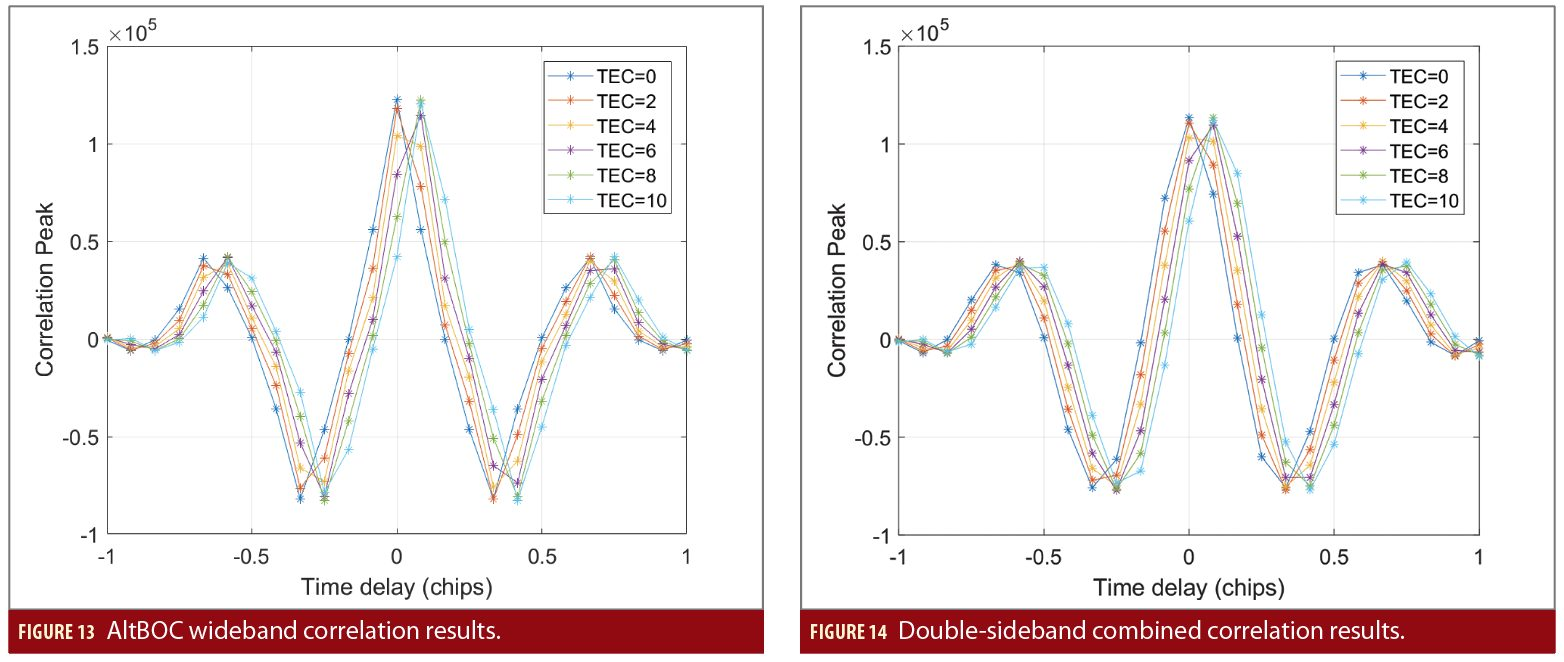(1) Tawk Y, Botteron C, Jovanovic A, et al. Analysis of Galileo E5 and E5ab code tracking[J]. GPS solutions, 2012, 16(2): 243-258.

(2) Ren J, Jia W, Chen H, et al. Unambiguous tracking method for alternative binary offset carrier modulated signals based on dual estimate loop[J]. IEEE communications letters, 2012, 16(11): 1737-1740.

(3) Zhu Y, Cui X, Lu M. Dual binary phase-shift keying tracking method for Galileo E5 AltBOC (15, 10) signal and its thermal noise performance[J]. IET Radar, Sonar & Navigation, 2015, 9(6): 669-680.

(4) Pany T, Falk N, Riedl B, et al. Wide-band signal processing features for reference station use of a PC-based software receiver: cross-correlation tracking on GPS L2P, AltBOC and the inter-frontend link for up to eight frequency bands[C]. ION GNSS 2011. 2011: 753-766.

(5) European Union, European GNSS (Galileo) open service signal in space interface control document, OS SIS ICD, Issue 2.0, January 2021.

### AuthorsJun Mo is CEO of GLOBSKY Technology Inc. He has a Masters degree in electronic engineering from Tsinghua University. He has more than 20 years industrial experience in GNSS receiver design and algorithm development. He currently provides professional consulting services on GNSS technologies.Tisheng Zhang is an associate professor of the GNSS Research Center at Wuhan University. He holds a Ph.D. in communication and information systems from Wuhan University. He was a post-doctoral student at Hong Kong Polytechnic University. His research interests focus on the fields of GNSS receiver and GNSS/INS deep integration.Xiaoji Niu is a professor in the GNSS Research Center at Wuhan University. He received a Ph.D. from Tsinghua University, did post-doctoral work at the University of Calgary and was a senior scientist a SiRF Technology. He leads a research group on GNSS/INS integration and low-cost navigation sensor fusion.